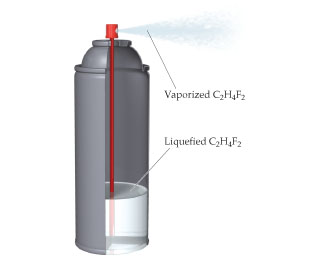# Problem: As shown here, one type of computer keyboard cleaner contains liquefied 1,1-difluoroethane (C2H4F2), which is a gas at atmospheric pressure. When the nozzle is squeezed, the 1,1-difluoroethane vaporizes out of the nozzle at high pressure, blowing dust out of objects.Defining the 1,1-difluoroethane as the system, do you expect qsys for the process to be positive or negative?

###### FREE Expert Solution

We are asked to determine if the qsys of 1,1-difluoroethane as the system is positive or negative.

The relationship between internal energy, heat, and work is shown in the following equation:

$\overline{){\mathbf{∆}}{\mathbf{U}}{\mathbf{=}}{\mathbf{q}}{\mathbf{+}}{\mathbf{w}}}$

ΔU = internal energy, J
q = heat, J
w = work, J

82% (344 ratings)###### Problem Details

As shown here, one type of computer keyboard cleaner contains liquefied 1,1-difluoroethane (C2H4F2), which is a gas at atmospheric pressure. When the nozzle is squeezed, the 1,1-difluoroethane vaporizes out of the nozzle at high pressure, blowing dust out of objects.Defining the 1,1-difluoroethane as the system, do you expect qsys for the process to be positive or negative?